从零开始_从零开始学java - CSDN

• 1. 激活函数激活函数这里选择最简单的sigmod（）函数
本文以实现逻辑回归为例，逻辑回归如图所示，只有一个神经元结点。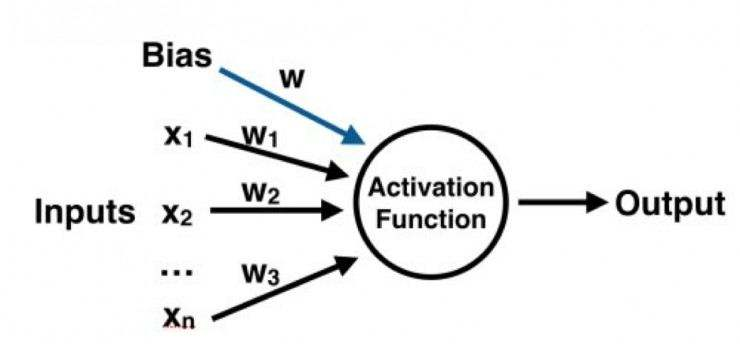1. 激活函数

logistic回归的激活函数一般使用sigmoid(x),其他情况可以使用tanh(x),ReLU(x)或者泄露ReLU(x),激活函数内容可以参考：从零开始搭建神经网络(一)基础知识。这里以sigmoid(x)为例表达式如下：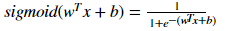def sigmoid(z):
"""
sigmoid激活函数
:param z: 输入
:return: sigmoid(z)
"""
return 1/(1 + np.exp(-z))

2.初始化参数

神经网络中有两个参数需要初始化分别是权重w和偏置b，初始化的方式可以全部初始化为0，也可以随机初始化。需要注意到的是对于logistic回归，可以将权重初始化为零，但是对于神经网络的歌参数数组全部初始化为零，再使用梯度下降那么就会无效。原因是无论哪个初始输入与零权重相乘的到的结果也全是零，从而激活函数输出也是一样的，最终导致反向传播的结果也是一样。由于本文实现的是逻辑回归，故采用零值初始化。

def initializeParamWithZeros(dim):
"""
初始化权重和偏置
:param dim: 输入维度
:return: 返回初始化的w和b
w:(dim,1)的向量
b:标量
"""
w = np.zeros((dim,1))
b = 0
return w,b


3.BP算法

BP算法分为两个部分：前向传播与反向传播。详细内容可以参考：从零开始搭建神经网络(一)基础知识。逻辑回归中前向传播用公式表示为：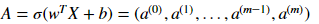其中X是输入数据，A是输出数据。反向传播是采用梯度下降法使误差函数减小，误差函数表示为：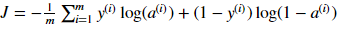其中m为输入数据数目，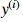是输入标签，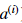是激活函数输出，即预测值。反向传播过程中使用梯度下降来实现损失函数的减小，需要先求得损失函数J对w和b的偏导分别为: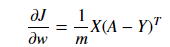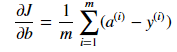def BackPropagate(w,b,X,Y):
"""
BP算法
:param w: 权重
:param b: 偏置
:param X: 输入数据
:param Y: 输入标签
:return: 梯度和损失函数
"""
#输入数据数目
num = Y.shape

#前向传播
A = sigmoid(np.dot(w.T,X) + b)
cost = -1 / num * np.sum(Y * np.log(A) + (1 - Y) * np.log(1 - A))

#反向传播
dw = 1 / num * np.dot(X,(A - Y).T)
db = 1 / num * np.sum(A - Y)

#用字典存储dw和db
"db": db}
return gradients,cost

4. 梯度下降与优化

神经网络的目标是针对参数W和b来求其损失函数J(W,b)的最小值，为了求解最优的W和b,我们可以重复梯度下降法的迭代步骤来求解最优的W和b，W和b更新公式为: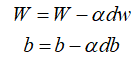其中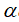为学习速率。

def Optimize(w,b,X,Y,iters,learning_rate):
"""
:param w: 初始权值
:param b: 初始偏置
:param X: 输入数据
:param Y: 输入数据标签
:param iters: 训练迭代次数
:param learning_rate: 学习速率
"""
#存储损失函数的值
costs = []
for i in range(iters):
#初始化梯度和损失函数的值

#获取偏导

#更新参数
w = w - learning_rate * dw
b = b - learning_rate * db

# 记录损失并输出函数
costs.append(cost)
print("The cost in the %d th iteration is %f" %(i,cost))

"db": db}
return w,b,gradients,costs

5.预测

训练好后的参数就可以在实际应用中使用了。预测结果分为两部分，第一部分计算输出：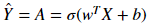然后判断输出和0.5的大小，大于0.5则为1，反之则为0

def predict(w,b,X):
"""
:param w: 训练后的权值
:param b: 训练后偏置
:param X: 输入数据
:return: 预测概率大于0.5的
"""
#获取输入数目m
m = X.shape
#存储预测结果
Y_prediction = np.zeros((1,m))
w = w.reshape(X.shape, 1)
#神经元输出
A = sigmoid(np.dot(w.T,X) + b)

#开始预测
for i in range(A.shape):
if A[0,i] > 0.5:
Y_prediction[0,i] = 1
else:
Y_prediction[0,i] = 0
return Y_prediction

6. 完整实例

import numpy as np
import matplotlib.pyplot as plt

if __name__ == "__main__":
#X为横坐标，Y为纵坐标
X = [0, 1.5, 2, 2, 2.25, 2.8, 3.2, 4]
Y = [1.5, 3, 4, 3, 0.5, 2.8, 1.35, 4]
label = [1, 1, 1, 1, 0, 0, 0, 0]
#第一类为蓝色，第二类为红色
label_color = ['blue','red']
color = []
for i in label:
if i == 1:
color.append(label_color)
else:
color.append(label_color)
for i in range(len(X)):
plt.scatter(X[i], Y[i], c = color[i])
plt.title('Raw Data')
plt.show()

#数据归一化
X = np.array(X)
Y = np.array(Y)
X = (X - np.average(X))
Y = (Y - np.average(Y))
X = X / X.max()
Y = Y / Y.max()
for i in range(len(X)):
plt.scatter(X[i], Y[i], c = color[i])
plt.title('Normalization Data')
plt.show()

data_X = np.vstack((X, Y))
data_label = np.array([label])
#参数设置
w = []
b = []
Y_prediction = []
iters = 20
learning_rate = 0.75
#开始训练
w,b = initializeParamWithZeros(data_X.shape)
w, b, gradients, costs = Optimize(w,b,data_X,data_label,iters,learning_rate)
Y_prediction = predict(w,b,data_X)

#画图
plt.plot(costs)
plt.ylabel('cost')
plt.xlabel('iterations')
plt.title("Learning rate =" + str(learning_rate))
plt.show()

#测试输入数据
point = input("Please enter a coordinates:\n")
#获取坐标
x = int(point.split(' '))
y = int(point.split(' '))
point_data = np.vstack((x,y))
point_prediction = predict(w,b,point_data)
print("The point is below to" ,end=" ")
print(point_prediction[0,0])

初始数据: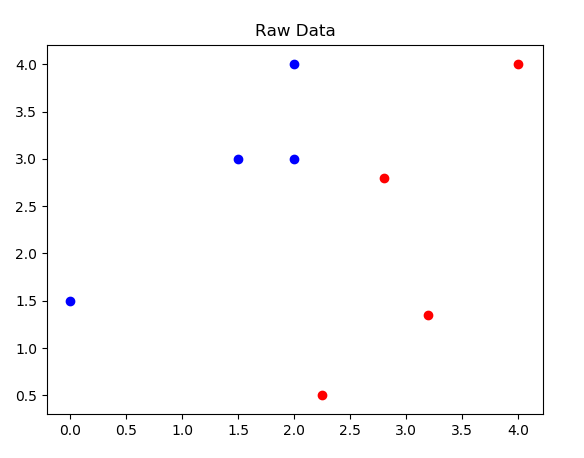归一化后的数据：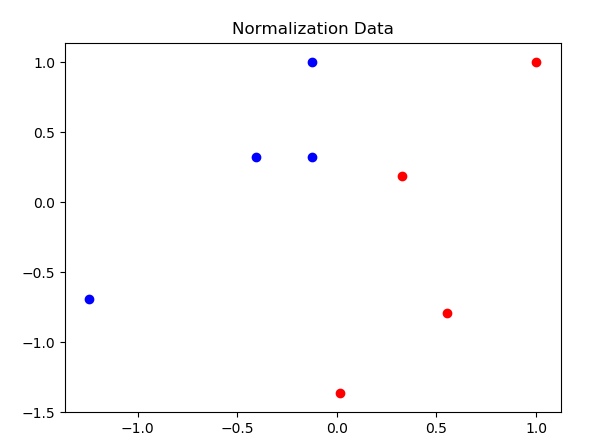损失函数随迭代次数的变化：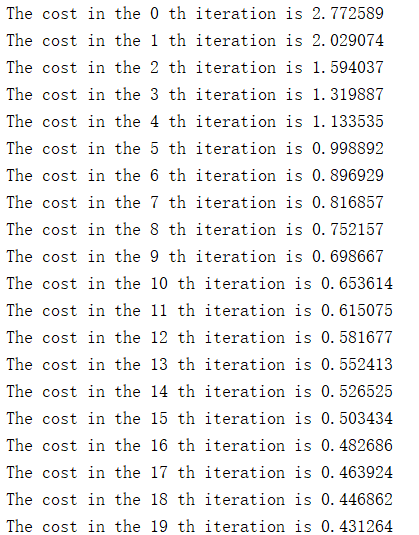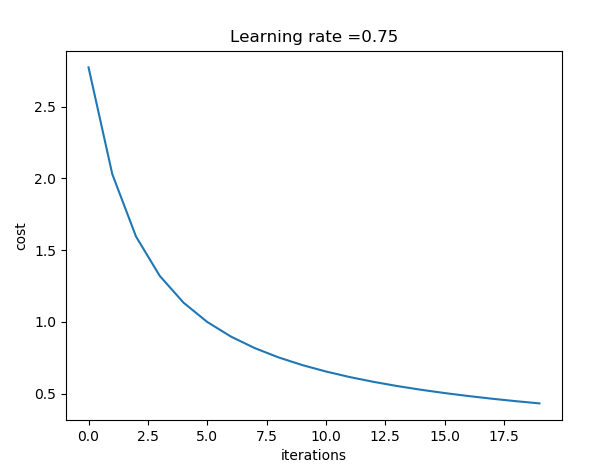输入一个坐标，进行回归分类：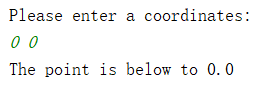展开全文• 目录 1.什么是人工神经网络 2.神经网络的相关概念 2.1 输入层、隐含层，输出层 2.2 激活函数 2.3 权重和偏置 ...神经网络起源于对生物神经元的研究，如下图所示生物神经元包括细胞体，树突，轴突等部分。...
目录

1.什么是人工神经网络

2.神经网络的相关概念

2.1 输入层、隐含层，输出层

2.2 激活函数

2.3 权重和偏置

2.4 损失函数

1.什么是人工神经网络

神经网络起源于对生物神经元的研究，如下图所示生物神经元包括细胞体，树突，轴突等部分。其中树突是用于接受输入信息，输入信息经过突触处理，当达到一定条件时通过轴突传出，此时神经元处于激活状态；反之没有达到相应条件，则神经元处于抑制状态。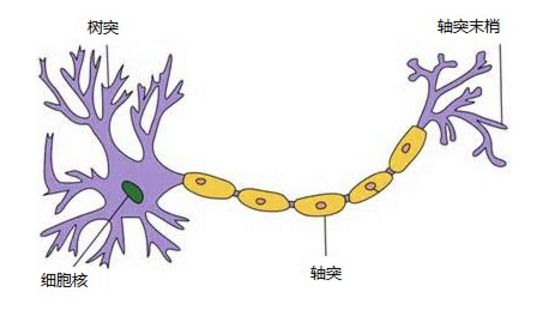受到生物神经元的启发，1943年心理学家McCulloch和数学家Pitts提出了人工神经元的概念，人工神经元又称为感知机，如下图所示。输入经过加权和偏置后，由激活函数处理后决定输出。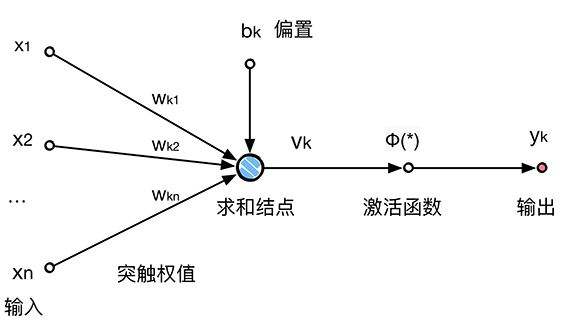其中生物神经元和人工神经元对应关系如下：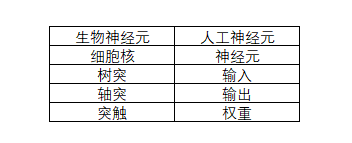由大量的人工神经元互相连接而形成的复杂网络结构成为人工神经网络（Artificial Neural Network, ANN）

2.神经网络的相关概念

2.1 输入层、隐含层，输出层

一般的神经网络包含以下几个部分，输入层，隐含层和输出层。下图是含有一个隐层的神经网络，隐层的层数越多，隐层结点数目越多，在非线性的激活函数下，神经网络就可以学习更深层次的特征。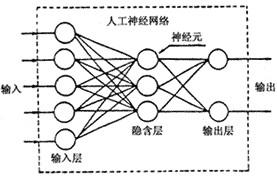2.2 激活函数

激活函数是神经网络设计的核心单元，激活函数是用来加入非线性因素的，因为线性模型的表达能力不够。激活函数需要满足一下几个条件：

1.非线性。如果激活函数是线性的，那么不管引入多少隐层，其效果和单层感知机没有任何差别。

2.可微性。训练网路时使用的基于梯度的优化方法需要激活函数必须可微。

3.单调性。单调性保证了神经网络模型简单。

几种常用的激活函数可以参考：点击打开链接

2.3 权重和偏置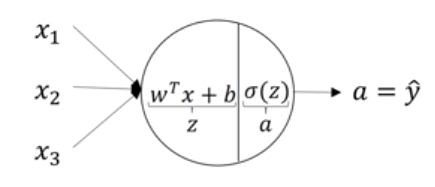如上图所示，假设神经元输入是X = [x1,x2,x3]，权重为w,偏置为b, 那么其输出为：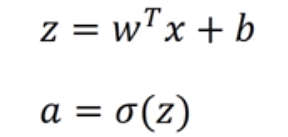可以看出,w，b会影响感知机的输出结果，正是因为此神经网络在迭代过程中修改的是权重w和偏置b。更细致的讲解可以参考：点击打开链接

2.4 损失函数

损失函数（loss function）也叫代价函数（cost function）。是神经网络优化的目标函数，神经网络训练或者优化的过程就是最小化损失函数的过程（损失函数值小了，对应预测的结果和真实结果的值就越接近）。损失函数也有很多种，拿常用的交叉熵损失函数举例，其单个样本二分类的损失函数公式如下：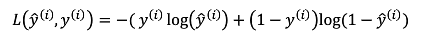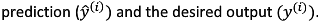当样本标签为1时，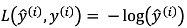，此时希望损失函数尽量的小，那么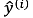就要接近1；

当样本标签为为0时，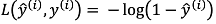，此时希望损失函数尽量的小，那么就要接近0。

对于m样本的损失函数可以使用：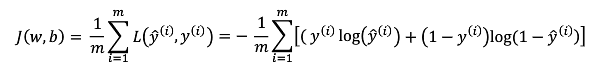其他的损失函数可以参考：点击打开链接

2.5 反向传播（Back Propagation,BP）算法

BP算法分为正向传播和误差的反向传播两个部分。
正向正向传播时，输入样本从输入层进入网络，经隐层逐层传递至输出层，如果输出层的实际输出与期望输出(导师信号)不同，则转至误差反向传播；如果输出层的实际输出与期望输出(导师信号)相同，结束学习算法。反向
反向反向传播时，将输出误差(期望输出与实际输出之差)按原通路反传计算，通过隐层反向，直至输入层，在反传过程中将误差分摊给各层的各个单元，获得各层各单元的误差信号，并将其作为修正各单元权值的根据。这一计算过程使用梯度下降法完成，在不停地调整各层神经元的权值和阈值后，使误差信号减小到最低限度。
权值和阈值不断调整的过程，就是网络的学习与训练过程，经过信号正向传播与误差反向传播，权值和阈值的调整反复进行，一直进行到预先设定的学习训练次数，或输出误差减小到允许的程度。

如图所示，其中蓝色为正向传播，红色为反向传播，反向传播的导数为损失函数对各变量求导。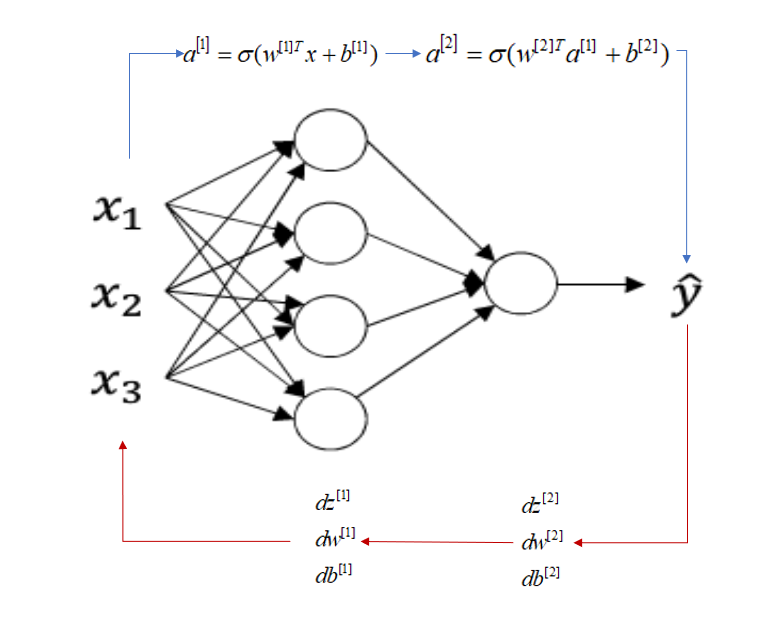展开全文•  单层感知机的学习能力有限，对于非线性可分的情况下分类效果不好，因此为了让网络拥有更强大的学习能力，需要在输入和输出层之间添加隐藏层。 下面实现一个简单含隐藏层的神经网络结构，是一个二分问题，输入...
 单层感知机的学习能力有限，对于非线性可分的情况下分类效果不好，因此为了让网络拥有更强大的学习能力，需要在输入和输出层之间添加隐藏层。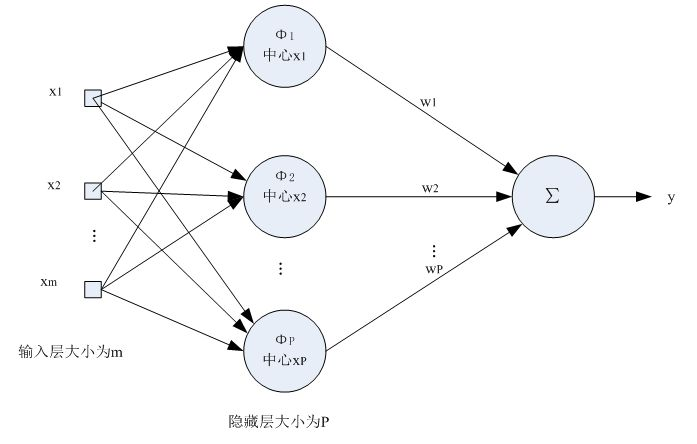下面实现一个简单含隐藏层的神经网络结构，是一个二分问题，输入为两维，输出为两类。

1.激活函数

由于添加了隐藏层，因此激活函数分为隐藏层的激活函数和输出层的激活函数，这里隐藏层的激活函数使用tanh()激活函数，输出层还是使用sigmoid()激活函数，这里使用上一篇的代码，至于tanh()激活函数到时候使用np.tanh()就行了

def sigmoid(z):
"""
sigmoid激活函数
:param z: 输入
:return: sigmoid(z)
"""
return 1/(1 + np.exp(-z))


2.初始化参数

初始化参数和不含隐藏层的升级网络没有多大区别，只是要初始化的参数多了一些而已，权重采用随机初始化，偏置零值初始化。这里需要讲一下各变量初始化的维度。以我们的图为例， 当处于在隐藏层这一层时，输入维度为（2，1），输出维度为（3，1），根据矩阵乘法公式和激活函数输出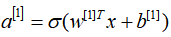，可以得到权重矩阵W的维度为（3，2），如下图所示。偏置的维度同理。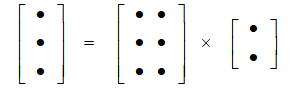def initialize_parameters(in_n, h_n, out_n):
"""
初始化权重和偏置
in_n -- 输入层节点数
h_n -- 隐藏层节点数
out_n -- 输出层结点数

returns:
params --
W1 --  权重矩阵，维度为(h_n, in_n)
b1 -- 偏置向量，维度为(h_n, 1)
W2 -- 权重矩阵，维度为 (out_n, h_n)
b2 -- 偏置向量，维度为 (out_n, 1)
"""
W1 = np.random.randn(h_n, in_n) * 0.01
b1 = np.zeros((h_n, 1))
W2 = np.random.randn(out_n, h_n) * 0.01
b2 = np.zeros((out_n, 1))

parameters = {"W1": W1,
"b1": b1,
"W2": W2,
"b2": b2}

return parameters

3.BP算法

含隐藏层的神经网络的BP算法要复杂一些因为要一层一层的正向传播，然后再反向传播回来。下面讲一下含隐藏层的BP算法。前向传播和不含隐藏层的神经网络一样，从输入层开始依次计算每一层的输入，上一次的输出当做当前层的输入；反向传播则是从输出层开始依次进行梯度下降，公式就不进行具体的推导了，在下面直接给出，可以参考：从零开始搭建神经网络（二）数学公式及代码实现。因为含隐藏层的BP算法比较复杂，所以前向传播和反向传播分开来写，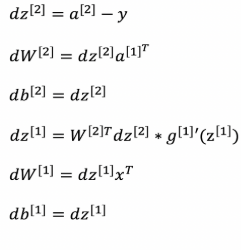def forward_propagation(X, parameters):
"""
前向传播
X -- 输入数据
parameters -- 参数（包含W1,b1,W2,b2）

returns:
A2 -- 网络输出
temp -- 包含 Z1, A1, Z2，A2的字典，用于BP算法
"""

W1 = parameters["W1"]
b1 = parameters["b1"]
W2 = parameters["W2"]
b2 = parameters["b2"]

Z1 = np.dot(W1, X) + b1
A1 = np.tanh(Z1)
Z2 = np.dot(W2, A1) + b2
A2 = sigmoid(Z2)

temp = {"Z1": Z1,
"A1": A1,
"Z2": Z2,
"A2": A2}
return A2,temp

def backward_propagation(parameters, temp, X, Y):
"""
反向传播
parameters -- 参数（包含W1,b1,W2,b2）
temp -- 包含 Z1, A1, Z2，A2的字典，用于BP算法
X -- 输入数据
Y -- 输入数据标签

returns:
cost -- 损失函数
"""
# 取回参数
W1 = parameters["W1"]
W2 = parameters["W2"]

A1 = temp["A1"]
A2 = temp["A2"]

# 样本数目
num = Y.shape
# 交叉熵损失函数
cost = -1 / num * np.sum(Y * np.log(A2) + (1 - Y) * np.log(1 - A2))

# 反向传播
dZ2 = A2 - Y
dW2 = 1 / num * np.dot(dZ2, A1.T)
db2 = 1 / num * np.sum(dZ2, axis=1, keepdims=True)
dZ1 = np.dot(W2.T, dZ2) * (1 - np.power(A1, 2))
dW1 = 1 / num * np.dot(dZ1, X.T)
db1 = 1 / num * np.sum(dZ1, axis=1, keepdims=True)

"db1": db1,
"dW2": dW2,
"db2": db2}

return cost,gradients

4.参数更新

参数更新和其他的完全一样，不懂的参考我上一篇博客。def update_parameters(parameters, gradients, learning_rate):
"""
梯度下降法更新参数

parameters -- 参数（包含W1,b1,W2,b2）

returns:
parameters -- 更新后的参数
"""
#取回参数
W1 = parameters["W1"]
b1 = parameters["b1"]
W2 = parameters["W2"]
b2 = parameters["b2"]

# 更新参数
W1 -= learning_rate * dW1
b1 -= learning_rate * db1
W2 -= learning_rate * dW2
b2 -= learning_rate * db2

parameters = {"W1": W1,
"b1": b1,
"W2": W2,
"b2": b2}

return parameters

5.预测

根据神经网络的输出值来进行预测，公式如下：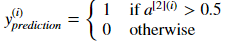def predict(parameters, X):
"""
使用学习好的参数来预测

parameters -- 学习好的参数
X -- 输入数据

Returns
predictions -- 预测结果0/1
"""
A2, temp = forward_propagation(X, parameters)
predictions = np.round(A2)

return predictions

6. 完整实例

下图是中的两类点是线性不可分的，利用这些数据测试下含隐藏层的神经网络的学习能力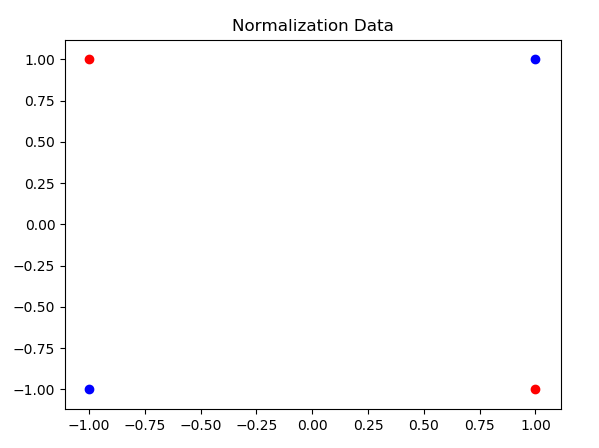if __name__ == "__main__":
# X为横坐标，Y为纵坐标
X = [0, 0, 1, 1]
Y = [0, 1, 0, 1]
label = [1, 0, 0, 1]
# 第一类为蓝色，第二类为红色
label_color = ['blue', 'red']
color = []
for i in label:
if i == 1:
color.append(label_color)
else:
color.append(label_color)

# 数据归一化
X = np.array(X)
Y = np.array(Y)
X = (X - np.average(X))
Y = (Y - np.average(Y))
X = X / X.max()
Y = Y / Y.max()
for i in range(len(X)):
plt.scatter(X[i], Y[i], c=color[i])
plt.title('Normalization Data')
plt.show()

data_X = np.vstack((X, Y))
data_label = np.array([label])

# 参数设置
in_n = 2
h_n = 3
out_n = 1
costs = []
Y_prediction = []
iters = 2000
learning_rate = 2
parameters = initialize_parameters(in_n, h_n, out_n)

#开始训练
for i in range(iters):
# 前向传播
A2, temp = forward_propagation(data_X, parameters)
# 反向传播
costTemp, gradients = backward_propagation(parameters, temp, data_X, data_label)
costs.append(costTemp)
# 参数更新

# 预测
Y_prediction = predict(parameters, data_X)

plot_decision_boundary(lambda x: predict(parameters, x.T), data_X, data_label)
plt.show()

# #画图
plt.plot(costs)
plt.ylabel('cost')
plt.xlabel('iterations')
plt.title("Learning rate =" + str(learning_rate))
plt.show()

分类结果如下图所示，可以发现含隐藏层的神经网络可以对线性不可分的数据进行分类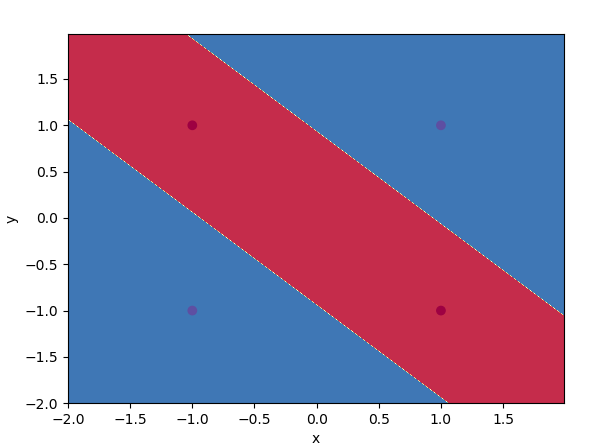P.S. 完整代码

import numpy as np
import matplotlib.pyplot as plt

def sigmoid(z):
"""
sigmoid激活函数
:param z: 输入
:return: sigmoid(z)
"""
return 1/(1 + np.exp(-z))

def initialize_parameters(in_n, h_n, out_n):
"""
初始化权重和偏置
in_n -- 输入层节点数
h_n -- 隐藏层节点数
out_n -- 输出层结点数
returns:
params --
W1 --  权重矩阵，维度为(h_n, in_n)
b1 -- 偏置向量，维度为(h_n, 1)
W2 -- 权重矩阵，维度为 (out_n, h_n)
b2 -- 偏置向量，维度为 (out_n, 1)
"""
W1 = np.random.randn(h_n, in_n) * 0.01
b1 = np.zeros((h_n, 1))
W2 = np.random.randn(out_n, h_n) * 0.01
b2 = np.zeros((out_n, 1))

parameters = {"W1": W1,
"b1": b1,
"W2": W2,
"b2": b2}

return parameters

def forward_propagation(X, parameters):
"""
前向传播
X -- 输入数据
parameters -- 参数（包含W1,b1,W2,b2）
returns:
A2 -- 网络输出
temp -- 包含 Z1, A1, Z2，A2的字典，用于BP算法
"""

W1 = parameters["W1"]
b1 = parameters["b1"]
W2 = parameters["W2"]
b2 = parameters["b2"]

Z1 = np.dot(W1, X) + b1
A1 = np.tanh(Z1)
Z2 = np.dot(W2, A1) + b2
A2 = sigmoid(Z2)

temp = {"Z1": Z1,
"A1": A1,
"Z2": Z2,
"A2": A2}
return A2, temp

def backward_propagation(parameters, temp, X, Y):
"""
反向传播
parameters -- 参数（包含W1,b1,W2,b2）
temp -- 包含 Z1, A1, Z2，A2的字典，用于BP算法
X -- 输入数据
Y -- 输入数据标签
returns:
cost -- 损失函数
"""
# 取回参数
W1 = parameters["W1"]
W2 = parameters["W2"]

A1 = temp["A1"]
A2 = temp["A2"]

# 样本数目
num = Y.shape
# 交叉熵损失函数
cost = -1 / num * np.sum(Y * np.log(A2) + (1 - Y) * np.log(1 - A2))

# 反向传播
dZ2 = A2 - Y
dW2 = 1 / num * np.dot(dZ2, A1.T)
db2 = 1 / num * np.sum(dZ2, axis=1, keepdims=True)
dZ1 = np.dot(W2.T, dZ2) * (1 - np.power(A1, 2))
dW1 = 1 / num * np.dot(dZ1, X.T)
db1 = 1 / num * np.sum(dZ1, axis=1, keepdims=True)

"db1": db1,
"dW2": dW2,
"db2": db2}

"""
梯度下降法更新参数
parameters -- 参数（包含W1,b1,W2,b2）
returns:
parameters -- 更新后的参数
"""
# 取回参数
W1 = parameters["W1"]
b1 = parameters["b1"]
W2 = parameters["W2"]
b2 = parameters["b2"]

# 更新参数
W1 -= learning_rate * dW1
b1 -= learning_rate * db1
W2 -= learning_rate * dW2
b2 -= learning_rate * db2

parameters = {"W1": W1,
"b1": b1,
"W2": W2,
"b2": b2}

return parameters

def predict(parameters, X):
"""
使用学习好的参数来预测
parameters -- 学习好的参数
X -- 输入数据
Returns
predictions -- 预测结果0/1
"""
A2, temp = forward_propagation(X, parameters)
predictions = np.round(A2)

return predictions

def plot_decision_boundary(model, X, y):
# 设置边界
x_min, x_max = X[0, :].min() - 1, X[0, :].max() + 1
y_min, y_max = X[1, :].min() - 1, X[1, :].max() + 1
h = 0.01

# 生成边界
xx, yy = np.meshgrid(np.arange(x_min, x_max, h), np.arange(y_min, y_max, h))
# 预测结构
Z = model(np.c_[xx.ravel(), yy.ravel()])
Z = Z.reshape(xx.shape)

# 画出边界
plt.contourf(xx, yy, Z, cmap=plt.cm.Spectral)
plt.ylabel('y')  # 标记y轴名称
plt.xlabel('x')  # 标记x轴名称
plt.scatter(X[0, :], X[1, :], c=np.squeeze(y), cmap=plt.cm.Spectral)

if __name__ == "__main__":
# X为横坐标，Y为纵坐标
X = [0, 0, 1, 1]
Y = [0, 1, 0, 1]
label = [1, 0, 0, 1]
# 第一类为蓝色，第二类为红色
label_color = ['blue', 'red']
color = []
for i in label:
if i == 1:
color.append(label_color)
else:
color.append(label_color)

# 数据归一化
X = np.array(X)
Y = np.array(Y)
X = (X - np.average(X))
Y = (Y - np.average(Y))
X = X / X.max()
Y = Y / Y.max()
for i in range(len(X)):
plt.scatter(X[i], Y[i], c=color[i])
plt.title('Normalization Data')
plt.show()

data_X = np.vstack((X, Y))
data_label = np.array([label])

# 参数设置
in_n = 2
h_n = 3
out_n = 1
costs = []
Y_prediction = []
iters = 2000
learning_rate = 2
parameters = initialize_parameters(in_n, h_n, out_n)

# 开始训练
for i in range(iters):
# 前向传播
A2, temp = forward_propagation(data_X, parameters)
# 反向传播
costTemp, gradients = backward_propagation(parameters, temp, data_X, data_label)
costs.append(costTemp)
# 参数更新

# 预测
Y_prediction = predict(parameters, data_X)

plot_decision_boundary(lambda x: predict(parameters, x.T), data_X, data_label)
plt.show()

# #画图
plt.plot(costs)
plt.ylabel('cost')
plt.xlabel('iterations')
plt.title("Learning rate =" + str(learning_rate))
plt.show()




展开全文• 虽然可以通过自己编程实现前向和反向传播过程但是随着神经网络的层数增加会导致编程趋于复杂，为了节省这种工作，可以使用现有深度学习框架。目前的已有的学习框架有很多Tensorflow,caffe,Torch,pytorch,Theano等，...
虽然可以通过自己编程实现前向和反向传播过程但是随着神经网络的层数增加会导致编程趋于复杂，为了节省这种工作，可以使用现有深度学习框架。目前的已有的学习框架有很多Tensorflow,caffe,Torch,pytorch,Theano等，使用最多的目前是Tensorflow,本文讲简单介绍下Tensorflow的使用方法。

1.预备工作

import tensorflow as tf
sess = tf.InteractiveSession()

第一行代码是导入tensorflow库，一般取别名为tf;第二行是创建一个新的InteractiveSession,使用这个命令会将这个session（会话）注册为默认的session,之后的运算都会在这个session里。

2.常量、变量、张量、占位符

Tensorflow中常量就是定以后值和维度都会变化的量，可以使数字或者数组。如下代码所示a是类型为tf.int16的数值，b是类型为tf.float32的数值，c是大小为4*4的零矩阵，矩阵元素类型为tf.float32

a = tf.constant(4, tf.int16)
b = tf.constant(8, tf.float32)
c = tf.constant(np.zeros(shape=(4,4), dtype=tf.float32))

变量就是数值可以变化的量,如下代码所示，如下代码所示a是类型为tf.int16的数值，b是类型为tf.float32的数值，c是大小为4*4的零矩阵，矩阵元素类型为tf.float32

a = tf.Variable(4, tf.int16)
b = tf.Variable(8, tf.float32)
c = tf.Variable(tf.zeros([4,4]))

TensorFlow用张量这种数据结构来表示所有的数据.你可以把一个张量想象成一个n维的数组或列表.一个张量有一个静态类型和动态类型的维数.张量可以在图中的节点之间流通，上面所说的a，b，c其实都是张量。

Tensorflow的占位符仅仅声明数据位置用于传入数据到计算图，占位符通过会话的feed_dict操作获取数据，形象理解就是一个萝卜一个坑，其中你每个样本的数据是萝卜，占位符就是萝卜坑。下面是占位符定义的例子,其中的None表示不用管行数。

x = tf.placeholder(tf.float32,[None,784])
y = tf.placeholder(tf.float32,[None,10])


3.参数初始化与网络结构

神经网络有许多参数，这些参数在训练之前需要初始化，Tensorflow中参数在定义的时候就可以初始化

w = tf.Variable(tf.zeros([784,10]))
b = tf.Variable(tf.zeros())

其他的参数如迭代次数和学习速率就像python普通定义变量一样

4.激活函数

Tensorflow的激活函数使用Tensorflow库提供的激活函数就可以了,以sigmoid函数为例，下面的代码对应着公式就是前向传播过程中结点的输出，在这个公式定义好后前向和反向内容都会自动实现。

y = tf.nn.sigmoid(tf.matmul(x,W) + b)

5.损失函数与优化器

Tensorflow也提供了许多损失函数和优化器，举个例子使用交叉熵作为损失函数，梯度下降作为优化算法.第一行中pred为神经网络输出的标签，y为真实标签；第二行learning_rate为学习速率。

loss = tf.reduce_mean(-tf.reduce_sum(pred * tf.log(y), reduction_indices=))
train_step = tf.train.GradientDescentOptimizer(learning_rate).minimize(loss)

6.训练

训练过程就是用优化器优化损失函数的过程，需要设置循环来进行迭代，举个例子,第一行是计算预测正确率，第二行是讲预测的正确率转换成tf.float32类型然后求平均，下面的for循环就是训练的主体，每迭代10次输出一次训练的正确率。值得说一下的是第三行代码，这行代码是一次性初始化所有变量；for循环中的第一行就是执行第5节中梯度下降优化算法来训练参数。（当训练数据较大时，会使用batch来进行批量训练）

correct_prediction = tf.equal(tf.argmax(y, 1), tf.argmax(pred, 1))
accuracy = tf.reduce_mean(tf.cast(correct_prediction, tf.float32), name='evaluation')

tf.global_variables_initializer().run()
epoch = 100
i = 0
for i in range(epoch):
train_step.run({x: train_x, pred: train_y})
if i % 10 == 0:
train_accuracy = accuracy.eval({x: train_x, pred: train_y})
print("epoch %d, train_accuracy %g" % (i, train_accuracy))

7.测试

测试就是使用训练好的参数在测试上计算神经网络的输出与真实标签之间的差异，很简单

test_accuracy = accuracy.eval({x:test_x, pred: test_y})

8.完整实例

下面是具有两层的神经网络，数据集采用MNIST，给了详细的注释

from tensorflow.example.tutorials.mnist import input_data
import tensorflow as tf
mnist = input_data.read_data_sets("MNIST_data/", one_hot = True) #导入数据，one-hot就是一行只有一个1，其余的全为0
sess.tf.InteractiveSession()

in_units = 784    #输入结点数
h1_units = 256    #隐含层结点数

#第一层的权重和偏置初始化，注意矩阵的维度
w1 = tf.Variable(tf.truncated_normal([in_units,h1_units]), stddev=0.1)
b1 = tf.Variable(tf.zeros([h1_units])
#第二层的权重和偏置初始化，注意矩阵的维度
w2 = tf.Variable(tf.truncated_normal([in_units,10]))
b2 = tf.Variable(tf.zeros()

# 占位符，keep-prob是神经网络为了防止过拟合采用dropout方法的概率
x = tf.placeholder(tf.float32,[None,in_units])
y = tf.placeholder(tf.float32,[None,10])
keep_prob = tf.placeholder(tf.float32)

hidden1 = tf.nn.relu(tf.matmul(x, w1) + b1              #第一层网络
hidden1_drop = tf.nn.dropout(hidden1, keep_prob)        #dropout
pred = tf.nn.softmax(tf.matmul(hidden_drop, w2) + b2)   #网络输出

loss = tf.reduce_mean(-tf.reduce_sum(pred * tf.log(y), reduction_indices=))    #损失函数

correct_prediction = tf.equal(tf.argmax(y, 1), tf.argmax(pred, 1))    #单个样本是否被正确分类，通过对比sotfmax输出最大的概率与真实标签
accuracy = tf.reduce_mean(tf.cast(correct_prediction, tf.float32), name='evaluation')    #计算全部样本的正确率

#开始训练
tf.global_variables_initializer().run()
epoch = 1000    #迭代次数为1000
i = 0
for i in range(epoch):
batch_xs,batch_xs = mnist.train.next_batch(100)    #采用mini-batch方法，每次随机抽取大小为100的数据
train_step.run({x: batch_xs, pred: batch_xs, keep_drop = 0.95})    #训练
if i % 100 == 0:    #每训练100次输出一次正确率
train_accuracy = accuracy.eval({x: train_x, pred: train_y, keep_drop = 0.95})
print("epoch %d, train_accuracy %g" % (i, train_accuracy))
#测试，输出模型在测试集上的正确率，测试时keep_drop要为1
print(accuracy.eval({x: mnist.test.images, pred: mnist.test.labels, keep_drop = 1.0}))


展开全文• 小白：师兄，g2o框架《从零开始一起学习SLAM | 理解图优化，一步步带你看懂g2o代码》，以及顶点《从零开始一起学习SLAM | 掌握g2o顶点编程套路》我都学完啦，今天给我讲讲g2o中的边吧！是不是也有什么套路？ 师兄：...计算机视觉
• ## 从零开始，一步一步编写网页

万次阅读 多人点赞 2016-10-08 15:39:03
1.编程工具WEB前端的学习环境非常简单，很多书上说，只需要PC上有浏览器和文本编辑器就成。本小白本着不怕死的冲劲试了试，还真是！！！（1）浏览器这个大家的PC都有吧？我的是360浏览器。（2）文本编辑器windows...javascript html5 css
• 从零开始系列从零开始玩htmlhtml从零开始玩css从零开始玩js从零开始玩git从零开始玩webpack从零开始玩vue从零开始玩react 从零开始玩html html html是前端的基础，里面封装了各式各样的标签，从古至今已经出现了上百...html css javascript
• 谁能明白我心呢？ 昨天已成过去，今天从头再来！不管成败与否，一切从零开始！ 转载于:https://www.cnblogs.com/lele/archive/2009/11/30/1613405.html...
• 作者: 曾宏远 / 崔荔蒙 出版社: 电子工业出版社 副标题: Android编程 出版年: 2012-10-1 页数: 265 ISBN: 9787121184925Android
• 从零开始写一个RTSP服务器系列 从零开始写一个RTSP服务器（一）不一样的RTSP协议讲解 从零开始写一个RTSP服务器（二）RTP传输H.264(待写) 从零开始写一个RTSP服务器（三）一个传输H.264的RTSP服务器(待写) 从零开始...rtsp协议 rtsp服务器
• “System.FormatException”类型的异常在 ...其他信息: 索引(从零开始)必须大于或等于零，且小于参数列表的大小。 去掉“，{2}” return string.Format("[{0},{1}]]", strb.ToString(), strData); 修改如下：
• 原因可能是代码中的占位符不匹配，或者没有按序号依次写好
• 1、Docker学习系列从零开始之centos6.5底下安装docker【一】 2、Docker学习系列从零开始之制作docker原始镜像【二】 3、Docker学习系列从零开始之启动镜像和进入相应的镜像【三】 4、Docker学习系列从零开始之...docker centos
• 今天我们从零开始搭建一个属于自己的博客网站，包括服务器，数据库等。 步骤分别为： 1、购买一台云服务器（我购买的阿里云服务器ECS，腾讯云，百度云服务器都是一样的） 2、购买域名（dingyinwu.com），然后...
• 只需要设置xAxis中的boundaryGap属性，设置为false代表是刻度开始，设置为true代表离刻度间隔一段距离 ... xAxis: { type: 'category', boundaryGap: false, data: dateList }, ...  ...Vue的使用
• rpc rpc 是基于 netty 实现的 java rpc 框架，类似于 dubbo。 主要用于个人学习，由渐入深，理解 rpc 的底层实现原理。 前言 工作至今，接触 rpc 框架已经有很长时间。 但是对于其原理一直只是知道个大概，从来没有...
• 从零开始开发一个大型网站 更新：前端代码已全部由TypeScript进行重写 这是本人第一个从零开始开发一个大型网站（前后端+部署代码），是一个内容分享社区，详细信息见github。目前还是开发中后期，随后还要进行...django vue fabric
• ## Kettle从入门到放弃，值得收藏

万次阅读 多人点赞 2018-07-12 10:04:08
【Kettle从零开始】一之Kettle简单介绍 http://blog.csdn.net/rotkang/article/details/20810921【Kettle从零开始】二之Kettle文件夹与界面介绍http://blog.csdn.net/rotkang/article/details/20875367【Kettle从零...
• ## Spring Boot 系列博客

万次阅读 2018-03-08 23:20:50
【Spring Boot 系列博客】 76. Spring Boot完美解决(406)Could not find ...75. Spring Boot 定制URL匹配规则【从零开始学Spring Boot】 71.mybatis 如何获取插入的id【从零开始学Spring Boot】 67. @Transac
• ## Spring Boot

万次阅读 2018-03-06 21:40:28
Spring Boot QQ交流群：193341332    【Spring Boot 系列博客】 76. Spring Boot完美解决(406)Could not find ...75. Spring Boot 定制URL匹配规则【从零开始学Spring Boot】    71.mybatis 如何获取插入的id
...